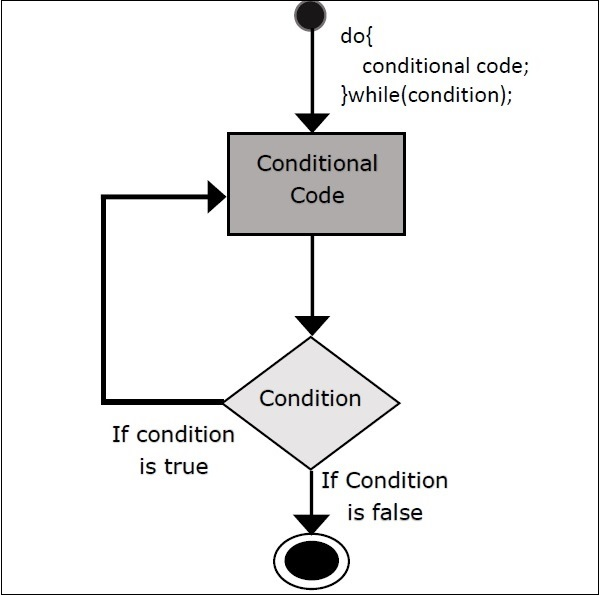# Scala - do-while Loop

Unlike while loop, which tests the loop condition at the top of the loop, the do-while loop checks its condition at the bottom of the loop. A do-while loop is similar to a while loop, except that a do-while loop is guaranteed to execute at least one time.

## Syntax

The following is the syntax for do-while loop.

```do {
statement(s);
}
while( condition );
```

Notice that the conditional expression appears at the end of the loop, so the statement(s) in the loop execute once before the condition is tested. If the condition is true, the flow of control jumps back up to do, and the statement(s) in the loop execute again. This process repeats until the given condition becomes false.

## Flow ChartTry the following example program to understand loop control statements (while statement) in Scala Programming Language.

## Example

```object Demo {
def main(args: Array[String]) {
// Local variable declaration:
var a = 10;

// do loop execution
do {
println( "Value of a: " + a );
a = a + 1;
}
while( a < 20 )
}
}
```

Save the above program in Demo.scala. The following commands are used to compile and execute this program.

## Command

```\>scalac Demo.scala
\>scala Demo
```

## Output

```value of a: 10
value of a: 11
value of a: 12
value of a: 13
value of a: 14
value of a: 15
value of a: 16
value of a: 17
value of a: 18
value of a: 19
```
scala_loop_types.htm